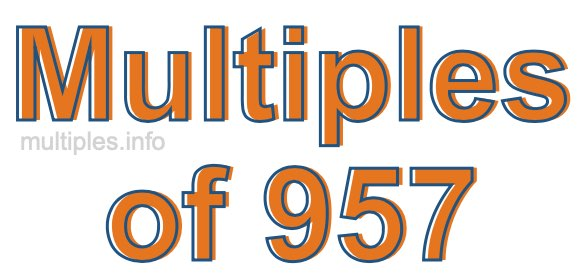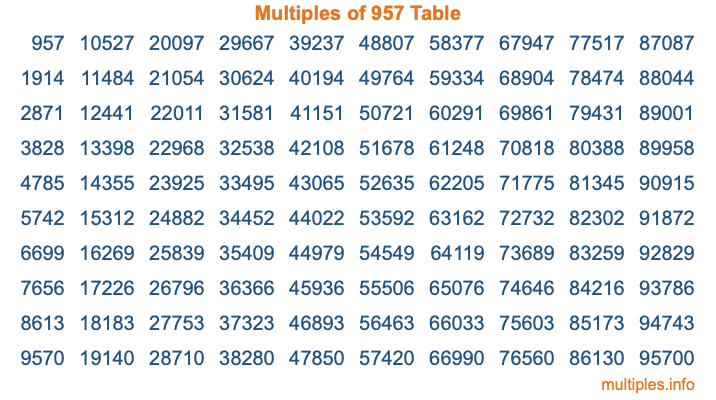Multiples of 957Welcome to the Multiples of 957 page. Here we will first teach you everything you will ever need to know about the multiples of 957, and then give you a study guide summary of everything we taught you to make sure you remember it all. Use this page to look up facts and learn information about the multiples of 957. This page will make you a multiples of nine hundred fifty-seven expert!

Definition of Multiples of 957
Multiples of 957 are all the numbers that when divided by 957 equal an integer. Each of the multiples of 957 are called a multiple. A multiple of 957 is created by multiplying 957 by an integer.

Therefore, to create a list of multiples of 957, you start with 1 multiplied by 957, then 2 multiplied by 957, then 3 multiplied by 957, and so on for as long as you want. Thus, the list of the first five multiples of 957 is 957, 1914, 2871, 3828, and 4785. To see a larger list of multiples of 957, see the printable image of Multiples of 957 further down on this page. We also have a category where you can choose any nth multiple of 957.

Multiples of 957 Checker
The Multiples of 957 Checker below checks to see if any number of your choice is a multiple of 957. In other words, it checks to see if there is any number (integer) that when multiplied by 957 will equal your number. To do that, we divide your number by 957. If the the quotient is an integer, then your number is a multiple of 957.

Is  a multiple of 957?

Least Common Multiple of 957 and ...
A Least Common Multiple (LCM) is the lowest multiple that two or more numbers have in common. This is also called the smallest common multiple or lowest common multiple and is useful to know when you are adding our subtracting fractions. Enter one or more numbers below (957 is already entered) to find the LCM.

Check out our LCM Calculator if you need more details about the Least Common Multiple or if you need the LCM for different numbers for adding and subtraction fractions.

nth Multiple of 957
As we stated above, 957 is the first multiple of 957, 1914 is the second multiple of 957, 2871 is the third multiple of 957, and so on. Enter a number below to find the nth multiple of 957.

th multiple of 957

Multiples of 957 vs Factors of 957
957 is a multiple of 957 and a factor of 957, but that is where the similarities end. All postive multiples of 957 are 957 or greater than 957. All positive factors of 957 are 957 or less than 957.

Below is the beginning list of multiples of 957 and the factors of 957 so you can compare:

Multiples of 957: 957, 1914, 2871, 3828, 4785, etc.

Factors of 957: 1, 3, 11, 29, 33, 87, 319, 957

As you can see, the multiples of 957 are all the numbers that you can divide by 957 to get a whole number. The factors of 957, on the other hand, are all the whole numbers that you can multiply by another whole number to get 957.

It's also interesting to note that if a number (x) is a factor of 957, then 957 will also be a multiple of that number (x).

Multiples of 957 vs Divisors of 957
The divisors of 957 are all the integers that 957 can be divided by evenly. Below is a list of the divisors of 957.

Divisors of 957: 1, 3, 11, 29, 33, 87, 319, 957

The interesting thing to note here is that if you take any multiple of 957 and divide it by a divisor of 957, you will see that the quotient is an integer.

Multiples of 957 Table
Below is an image of the first 100 multiples of 957 in a table. The table is in chronological order, column by column. The first column has the first ten multiples of 957, the second column has the next ten multiples of 957, and so on.The Multiples of 957 Table is also referred to as the 957 Times Table or Times Table of 957. You are welcome to print out our table for your studies.

Negative Multiples of 957
Although not often discussed or needed in math, it is worth mentioning that you can make a list of negative multiples of 957 by multiplying 957 by -1, then by -2, then by -3, and so on, to get the following list of negative multiples of 957:

-957, -1914, -2871, -3828, -4785, etc.

Multiples of 957 Summary
Below is a summary of important Multiples of 957 facts that we have discussed on this page. To retain the knowledge on this page, we recommend that you read through the summary and explain to yourself or a study partner why they hold true.

There are an infinite number of multiples of 957.

A multiple of 957 divided by 957 will equal a whole number.

957 divided by a factor of 957 equals a divisor of 957.

The nth multiple of 957 is n times 957.

The largest factor of 957 is equal to the first positive multiple of 957.

957 is a multiple of every factor of 957.

957 is a multiple of 957.

A multiple of 957 divided by a divisor of 957 equals an integer.

957 divided by a divisor of 957 equals a factor of 957.

Any integer times 957 will equal a multiple of 957.

Multiples of a Number
Here you can get the multiples of another number, all with the same attention to detail as we did for multiples of 957 on this page.

Multiples of
Multiples of 958
Did you find our page about multiples of nine hundred fifty-seven educational? Do you want more knowledge? Check out the multiples of the next number on our list!